# Irodov Solutions: Properties of Atoms. Spectra- 2 Notes | Study I. E. Irodov Solutions for Physics Class 11 & Class 12 - JEE

## JEE: Irodov Solutions: Properties of Atoms. Spectra- 2 Notes | Study I. E. Irodov Solutions for Physics Class 11 & Class 12 - JEE

The document Irodov Solutions: Properties of Atoms. Spectra- 2 Notes | Study I. E. Irodov Solutions for Physics Class 11 & Class 12 - JEE is a part of the JEE Course I. E. Irodov Solutions for Physics Class 11 & Class 12.
All you need of JEE at this link: JEE

Q.116. Find the degeneracy of the states 2P, 3D, and 4F possessing the greatest possible values of the total angular momentum.

Ans. The state with greatest possible total angular momentum are

For a 2P state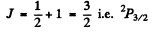Its degeneracy is 4.

For a 3D state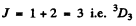Its degeneracy is 2 x 3 + 1 = 7

For a 4F state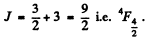Its degeneracy is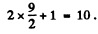Q.117. Write the spectral designation of the term whose degeneracy is equal to seven and the quantum numbers L and S are interrelated as L = 3S.

Ans. The degeneracy is 2J + 1. So we must have J = 3. From L = 3 S, we see that S must be an integer since L is integral and S can be either integral or half integral. If S = 0 then L = 0 but this is consistent with J = 3. For  S ≥ 2, L ≥ 6 and then J = 3. Thus the state is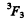Q.118. What element has the atom whose K, L, and M shells and 4s subshell are filled completely and 4p subshell is half-filled?

Ans. The order of filling is

K , L , M shells, then 4 s2 , 3d10 then 4p3. The electronic configuration of the element will be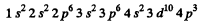(There must be three 4p electrons)

The number of electrons is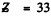and the element is As. (The 3d subshell must be filled before 4p fills up.)

Q.119. Using the Hund rules, find the basic term of the atom whose partially filled subshell contains (a) three p electrons; (b) four p electrons.

Ans. (a) when the partially filled shell contains three p electrons, the total spin S must equal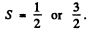The state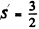has maximum spin and is totalIy symmetric under exchange of spin lables. By Pauli’s exclusion principle this implies that the angular part of the wavefunction must be totally anti symmetric. Since the angular part of the wave function a p electron is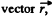the total wavefunction of three p electrons is the totally antisymmetric combination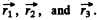The only such combination is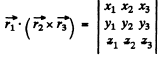This combination is a scalar and hence has L = 0. The spectral term of the ground state is then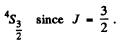(b) We can think of four p electrons as consisting of a full p shell with two p holes. The state of maximum spin S is then S = 1. By Pauli’s principle the orbital angular momentum part must be antisymmetric and can only have the form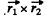where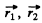are the coordinates of holes. The result is harder to see if we do not use the concept of holes. Four p electrons can have S = 0, 1, 2 but the S = 2 state is totally symmetric. The corresponding angular wavefunction must be totally antisymmetric. But this is impossible : there is no quantity which is amtisymmetric in four vectors. Thus the maximum allowed S is S = 1. We can construct such a state by coupling the spins of electrons 1 & 2 to 5 = 1 and of electrons 3 & 4 to S = 1 and then coupling the resultant spin states to S = 1. Such a state is symmetric under the exchange of spins of 1 & 2nd 3 and 4 but antisymmetric under the simultaneous exchange of (1, 2) & (3, 4). the conjugate angular wavefimction must be antisymmetric under the exchange of (1, 2) and under the exchange of (3, 4) by Pauli principle. It must also be antisymmetric under the simultaneous exchange of (1, 2) and (3, 4). (This is because two exchanges of electrons are involved.) The required angular wavefunction then has the formand is a vector, L = 1 . Thus, using also the fact that the shell is more than half full, we find the spectral term 3P2

( J = L + S ) .

Q.120. Using the Hund rules, find the total angular momentum of the atom in the ground state whose partially filled subshell contains (a) three d electrons; (b) seven d electrons.

Ans. (a) The maximum spin angular momentum of three electrons can be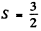. This state is totally symmetric and hence the conjugate angular wavefunction must be antisymmetric By Pauli’s exclusion principle the totally antisymmetric state must have different magnetic quantum numbers. It is easy to see that for d electrons the maximum value of the magnetic quantum number for oibital angular momentum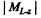= 3 (from 2 + 1 + 0). Higher values violate Pauli’s principle. Thus the state of highest orbital angular momentum consistent with Pauli’s principle is L = 3.

The state of the atom is then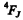where J = L - S by H und’s rule. Thus we get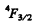The magnitude of the angular momentum is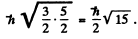(b) Seven d electrons mean three holes. Then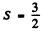and L = 3 as before. But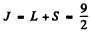by Hund’s rule for more than half filled shell. Thus the state is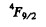Total angular momentum has the magnitude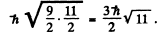Q.121. Making use of the Hund rules, find the number of electrons in the only partially filled subshell of the atom whose basic term is (a) 3F2; (b) 2P3/2; (c) 6S5/2

Ans. (a)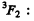The maximum value of spin is S = 1 here. This means there are 2 electrons.
L - 3 so s and p electrons are ruled out. Thus the simplest possibility is d electrons. This is the correct choice for if we were considering f electrons, the maximum value of L allowed by Pauli principle will be L = 5 (maximum value of the magnitude of magnetic quantum number will be 3 + 2 - 5 .)

Thus the atom has two d electrons in the unfilled shell.

(b)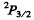Here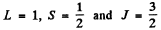Since J = L + S, Hund’s rule im plies the shell is more than halffull. This means one electron less than a full shell. On the basis of hole picture it is easy to see that we have p electrons. Thus the atom has 5 p electrons.

(c)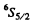Here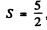L = 0 . We either have five electrons or five holes. The angular part is antisymmetric. For five d electrons, the maximum value of the quantum number consistent with Pauli exclusion principle is ( 2 + l + 0 - l - 2 ) = 0 so L = 0 . For f or g electrons L > 0 whether the shell has five electrons or five holes. Thus the atom has five d electrons.

Q.122. Using the Hund rules, write the spectral symbol of the basic term of the atom whose only partially filled subshell (a) is filled by 1/3, and S = 1; (b) is filled by 70%, and S = 3/2.

Ans. (a) If S = 1 is the maximum spin then there must be two electrons (If there are two holes then the shell will be more than half full.). This means that there are 6 electrons in the full shell so it is a p shell. By Paul’s principle the only antisymmetric combination of two electrons has L = 1 Also J = L - S as the shell is less than half full. Thus the term is 3P

(b)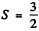means either 3 electrons or 3 holes. As the shell is more than half full the former possibility is ruled out. Thus we must have seven d electrons. Then as in problem 6.120 we get the term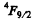Q.123. The only partially filled subshell of a certain atom contains three electrons, the basic term of the atom having L = 3. Using the Hund rules, write the spectral symbol of the ground state of the given atom.

Ans. With three electrons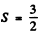and the spin part is totally symmetric. It is given that the basic term has L = 3 so L = 3 is the state o f highest orbital angular momentum. This is not possible with p electron so we must have d electrons for which L = 3 for 3 electrons. For three f, g electrons L > 3. Thus we have 3 d electrons. Then as in (6.120) the ground state is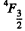Q.124. Using the Hund rules, find the magnetic moment of the ground state of the atom whose open subshell is half-filled with five electrons.

Ans. We have 5d electrons in the only unfilled shell. Then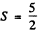aximum value of L consistent with Pauli’s principle is L = 0. Then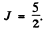So by Lande’s formula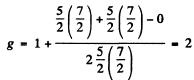Thus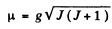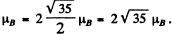The ground state is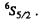Q.125. What fraction of hydrogen atoms .is in the state with the principal quantum number n = 2 at a temperature T = 3000 K?

Ans. By Boltzmann formula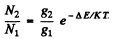Here ΔE = energy difference between n = 1 and n = 2 states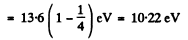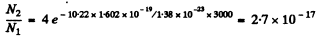Explicitly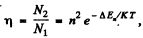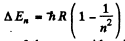for the nth excited state because the degeneracy of the state with principal quantum number n is 2 n2.

Q.126. Find the ratio of the number of atoms of gaseous sodium in the state 3P to that in the ground state 3S at a temperature T 2400 K. The spectral line corresponding to the transition 3P → 3S is known to have the wavelength λ = 589 nm.

Ans. We have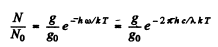Here g = degeneracy ofthe 3P sate = 6, g0 = degeneracy of the 3S state = 2 and λ  wavelength of the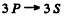line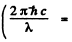energy difference between 3P & 3S levels.

Substitution gives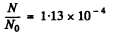Q.127. Calculate the mean lifetime of excited atoms if it is known that the intensity of the spectral line appearing due to transition to the ground state diminishes by a factor η = 25 over a distance l = 2.5 mm along the stream of atoms whose velocity is v = = 600 m/s.

Ans. Let T = mean life time o f the excited atoms. Then the number o f excited atoms will decrease with time as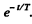In time t the atom travels a distance vt so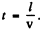Thus the number of excited atoms in a beam that has traversed a distance l has decreased by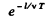The intensity of the line is proportional to the number of excited atoms in the, beam. Thus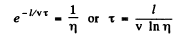= 1.29 x 10-6 second.

Q.128. Rarefied Hg gas whose atoms are practically all in the ground state was lighted by a mercury lamp emitting a resonance line of wavelength λ = 253.65 nm. As a result, the radiation power of Hg gas at that wavelength turned out to be P = 35 mW. Find the number of atoms in the state of resonance excitation whose mean lifetime is ζ = 0.15μs.

Ans. As a result of the lighting by the mercury lamp a number of atoms are pumped to the excited state. In equilibrium the number of such atoms is N. Since the mean life time of the atom is T, the number decaying per unit time is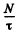Since a photon of energy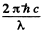results from each decay, the total radiated power will be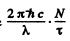. This must equal P. Thus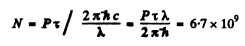Q.129. Atomic lithium of concentration n = 3.6.1016  cm-3  is at a temperature T = 1500 K. In this case the power emitted at the resonant line's wavelength λ = 671 nm (2P → 2S) per unit volume of gas is equal to P = 0.30 W/cm3. Find the mean lifetime of Li atoms in the resonance excitation state.

Ans. The number of excited atoms per unit volume of the gas in 2P state is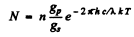Here gp = degeneracy of the 2p state = 6, gr = degeneacy of the 2s state = 2 and λ = wavelength of the resonant line 2 p → 2 s . The rate of decay of these atoms isper sec. per unit volume. Since each such atom emits light of wavelength X, we must have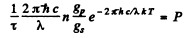Thus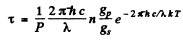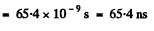Q.130. Atomic hydrogen is in thermodynamic equilibrium with its radiation. Find: (a) the ratio of probabilities of induced and spontaneous radiations of the atoms from the level 2P at a temperature T = 3000 K; (b) the temperature at which these probabilities become equal.

Ans. (a) We know that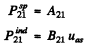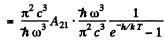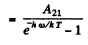Thus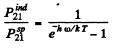For the transition 2P→1S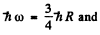we get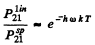substitution gives 7 x 10-18

(b) The two rates become equal when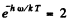or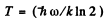= 1.71 x 10K

The document Irodov Solutions: Properties of Atoms. Spectra- 2 Notes | Study I. E. Irodov Solutions for Physics Class 11 & Class 12 - JEE is a part of the JEE Course I. E. Irodov Solutions for Physics Class 11 & Class 12.
All you need of JEE at this link: JEEUse Code STAYHOME200 and get INR 200 additional OFF

## I. E. Irodov Solutions for Physics Class 11 & Class 12

111 docs

Track your progress, build streaks, highlight & save important lessons and more!

,

,

,

,

,

,

,

,

,

,

,

,

,

,

,

,

,

,

,

,

,

;